# Football Players

Twelve football players at Lincoln High School are training to gain a healthy weight and strength before their first game. Pete’s Restaurant will have a dinner for the football players if their combined weight totals at least two thousand pounds. At the end of training, the coach weighs and records the weight of each player with a very precise digital scale. Below is the weight of each football player:

 Player Weight in Pounds 1 162.090 2 182.300 3 139.865 4 197.500 5 199.980 6 194.231 7 200.900 8 145.700 9 181.200 10 149.970 11 198.909 12 157.310

The coach rounds each football player's weight to the nearest hundred pounds to estimate if the football players earn dinner at Pete's Restaurant. Will the football players be going to Pete's Restaurant for dinner? The football players look at the coach's list of precise weights. The football players decide to make a new list with each football players weight recorded to the nearest tenth of a pound. What could the new list look like? Show all your mathematical thinking.

Assessment

## Plan

### Suggested materials

Engagement Image:

Teachers may project the image below to launch this task for their students, define nouns, promote discussion, access prior knowledge, and inspire engagement and problem solving.

## Solutions tabs

### Expert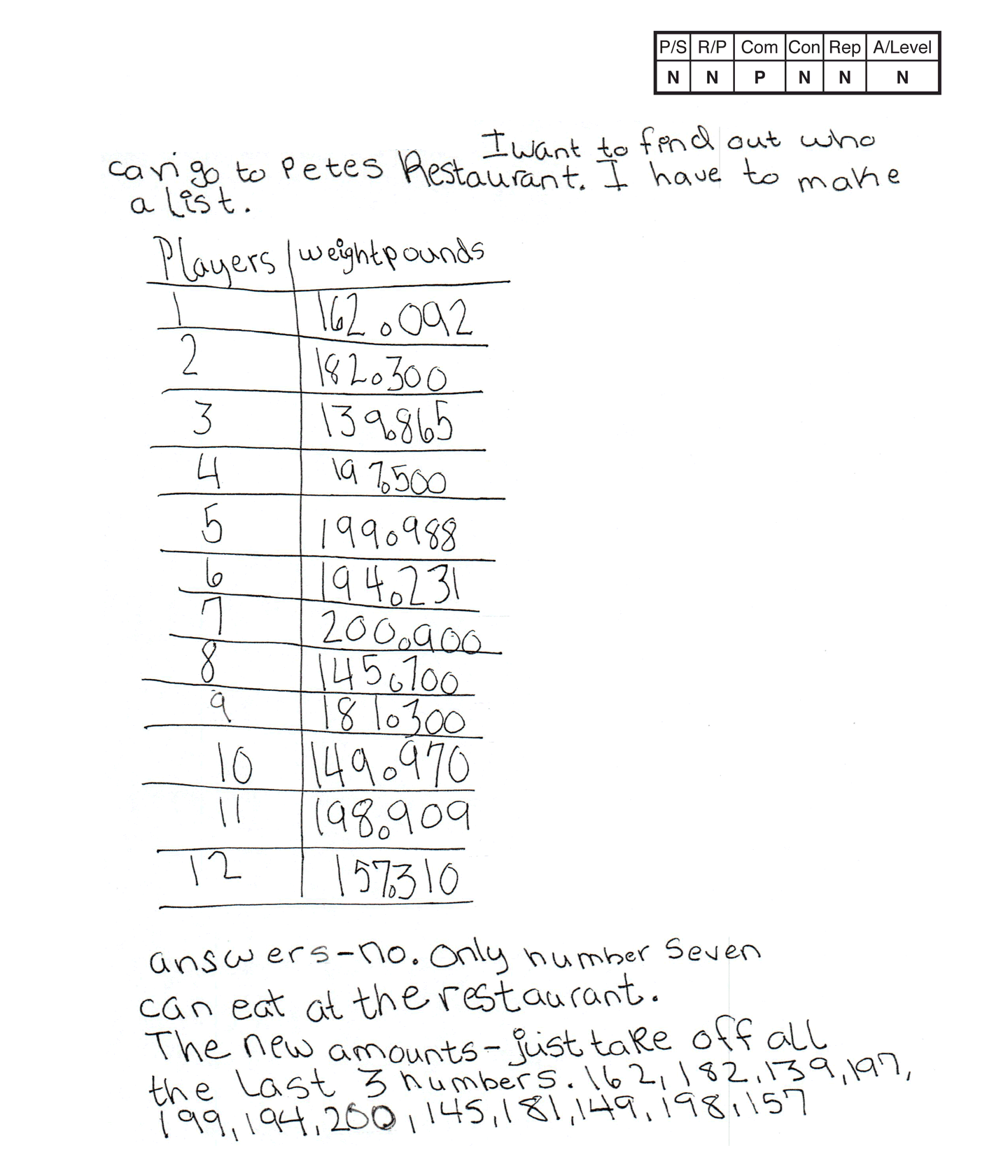This student does not meet the standard.

### Scoring Rationale

#### Novice

The student's strategy of copying the coach's list of players and their weights in pounds would not work to solve the first part of the task. The student's answer, "No, only number seven can eat at the restaurant," is not correct. The student's strategy, "just take off all the last 3 numbers," would not work to solve the second part of the task. The student's answer, "162, 182, 139, 197, 199, 194, 200, 145, 181, 149, 198, 157" is not correct.

#### Novice

The student does not demonstrate correct reasoning and proof of the underlying concepts of the task. The student simply copies the coach's list of each player's weight and selects the seventh football player because the player weighs the most in the list. The student does not show understanding of rounding/estimating, and understanding that a total of the players' weights is needed. The student's reasoning for the second part of the task seems to be that rounding means to list the numbers to the left of the decimal point.

#### Practitioner

The student correctly uses the mathematical terms weights, pounds from the task. The student also uses the terms number, amount. The student correctly uses decimal notation to indicate the weight of player 2, 3, 4, 6, 7, 8, 10, 11, 12.

#### Novice

The student solves the task and does not make a mathematically relevant observation.

#### Novice

Copying the list of the football players and their weights in pounds given in the task is not considered an independent attempt at making a representation. The copied list also has incorrect data listed for players one, five, and nine.

#### Novice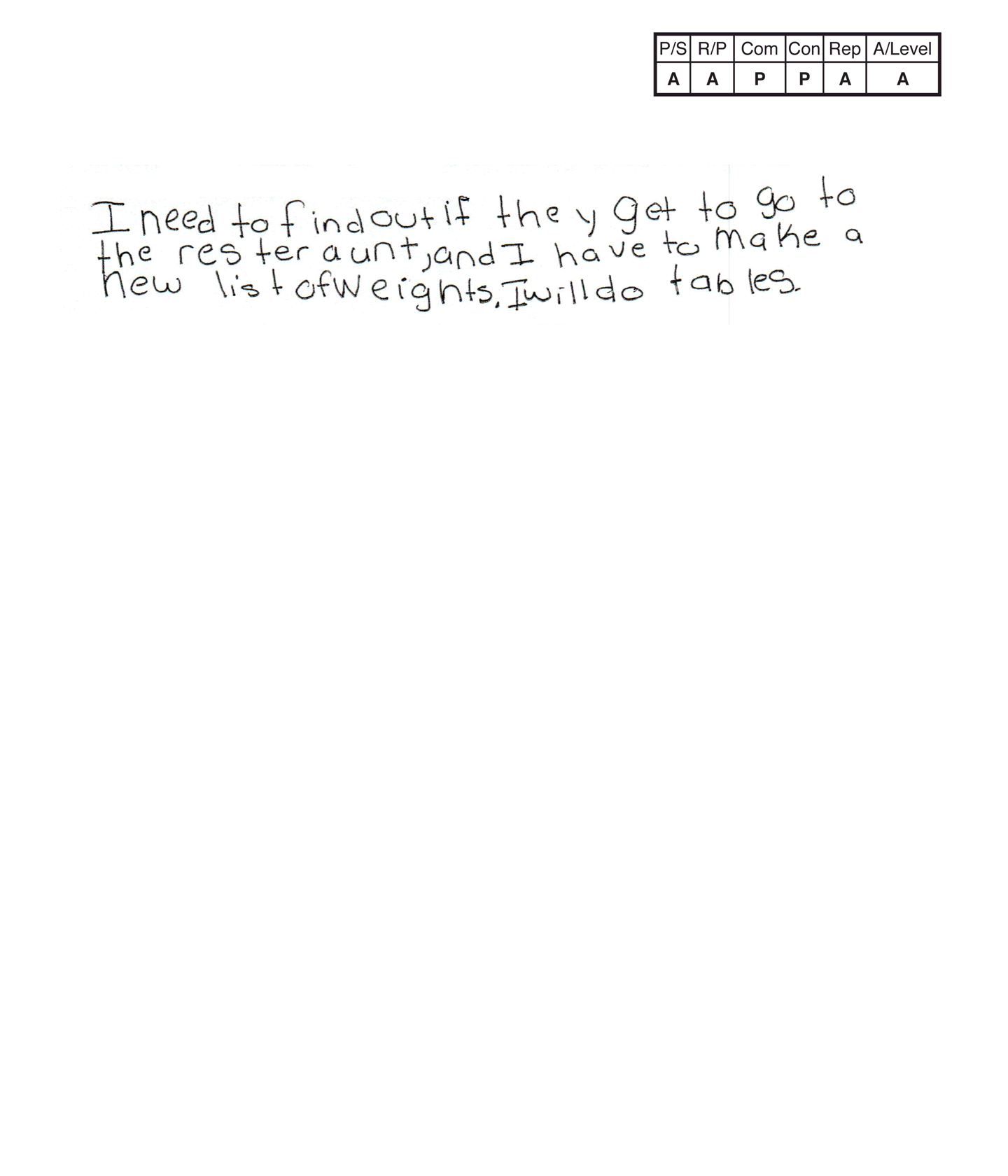,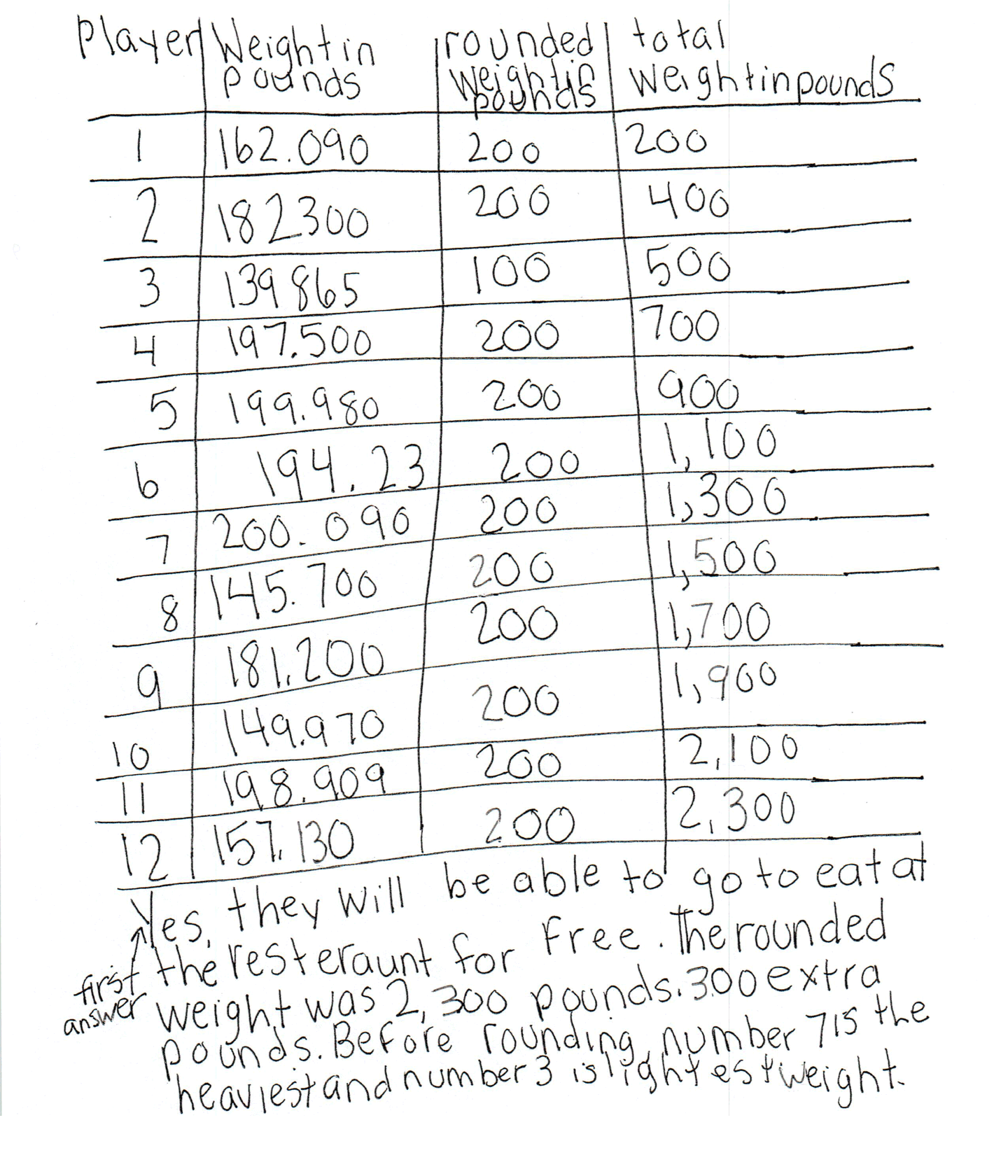,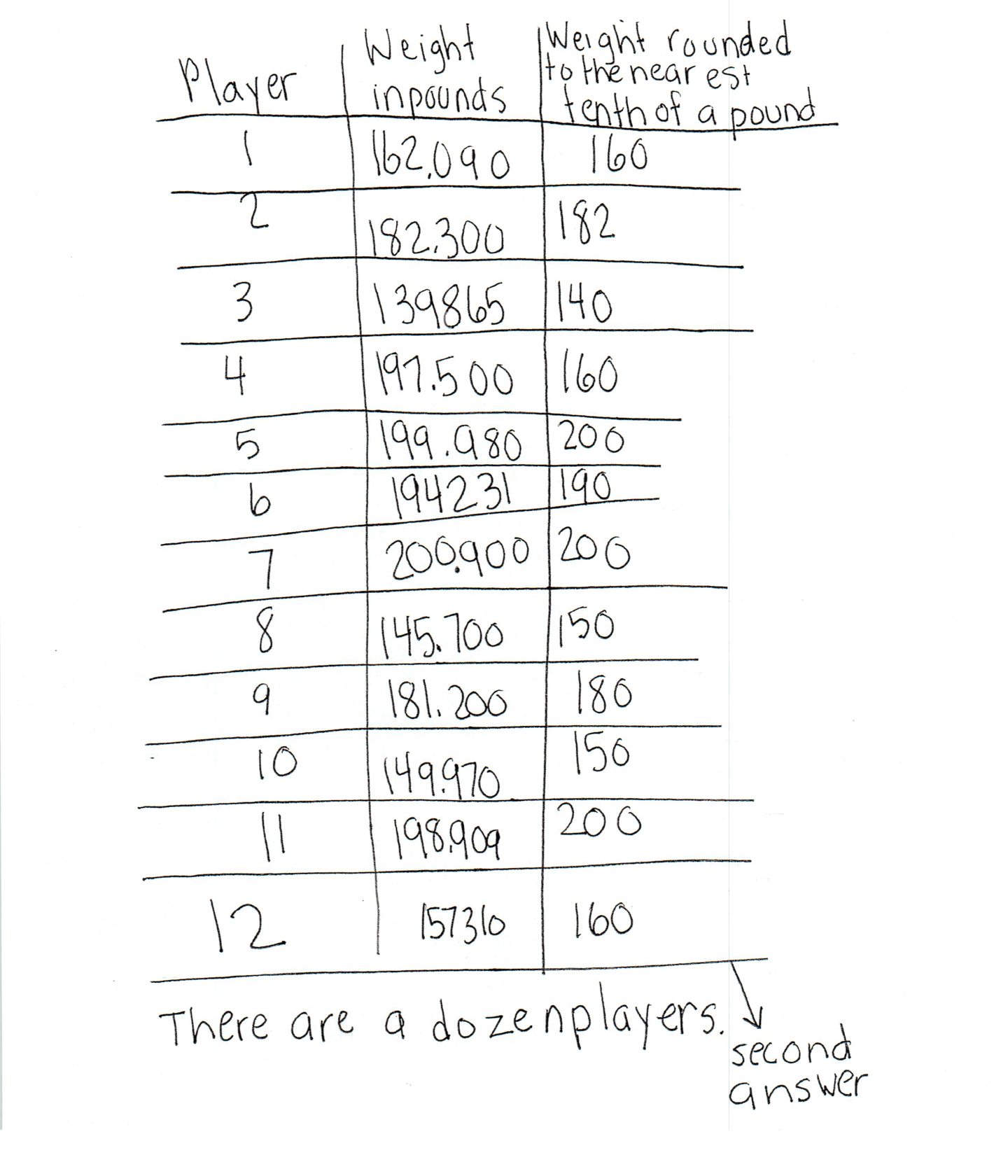This student does not meet the standard.

### Scoring Rationale

#### Apprentice

The student's strategy of using a table to indicate each football player's weight and that weight rounded to the nearest 100 would work to solve the first part of the task. The student does not correctly notate the eighth and tenth player's actual weight which leads to an incorrect total weight in pounds. The students answer, "Yes, they will be able to go to eat at the resteraunt for free," is determined on an incorrect total estimated weight. The student's strategy of using a table to indicate each player's weight rounded to the nearest tenth of a pound would work to solve the task but it appears the student rounds each number to the nearest 10 instead of tenth which results in a correct answer for only players 5 and 10.

#### Apprentice

The student demonstrates understanding of some of the underlying concepts of the task. The student notes the exact weight of each football player and correctly rounds all but two weights to the nearest 100. The student shows correct reasoning in adding the rounded weights to compare the sum to 2,000 pounds. The student does not demonstrate understanding of rounding to the nearest tenth. It appears that the student rounds each player’s weight to the nearest 10.

#### Practitioner

The student correctly uses the mathematical terms weights, pounds from the task. The student does not earn credit for the term tenth, because the student did not round to the nearest tenth. The student also uses the terms table, total, first, second, dozen. The student correctly uses the decimal notation for most of the player's weights.

#### Practitioner

The student makes the mathematically relevant observations, "Before rounding, number 7 is the heaviest and number 3 is the lightest weight," and "There are a dozen players."

#### Apprentice

The students first table is appropriate to the task but is not accurate. The decimal point is missing in the "Weight in pounds" column for players two and three. The "rounded weights in pounds" is incorrect for players eight and 10 which results in the "total weight in pounds" column being incorrect after player seven. The student's second table is appropriate to the task but is not accurate. The decimal point is missing in the "Weights in pounds" for players 3, 6, and 12. The recorded data for "Weight rounded to the nearest tenth of a pound" is not correct.

#### Apprentice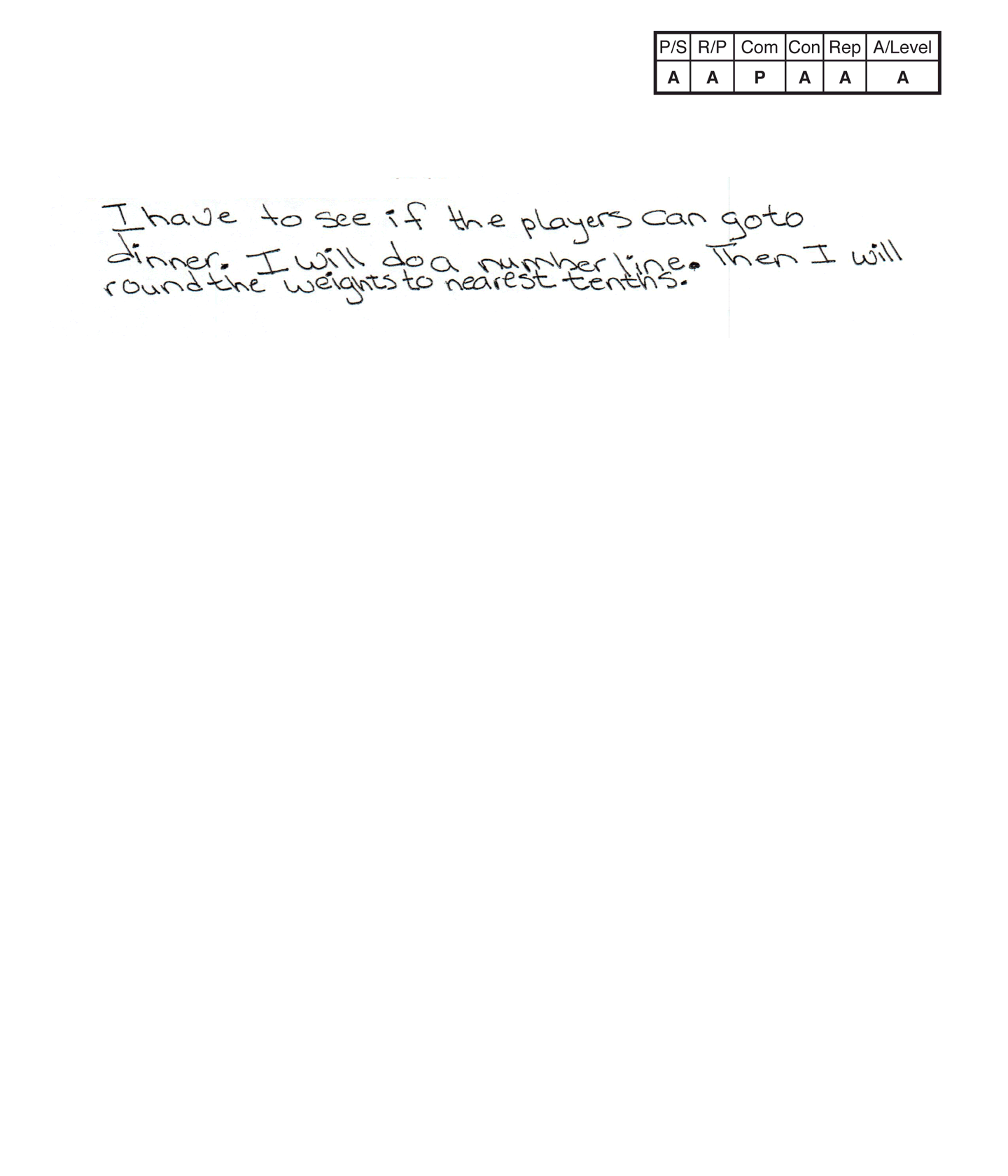,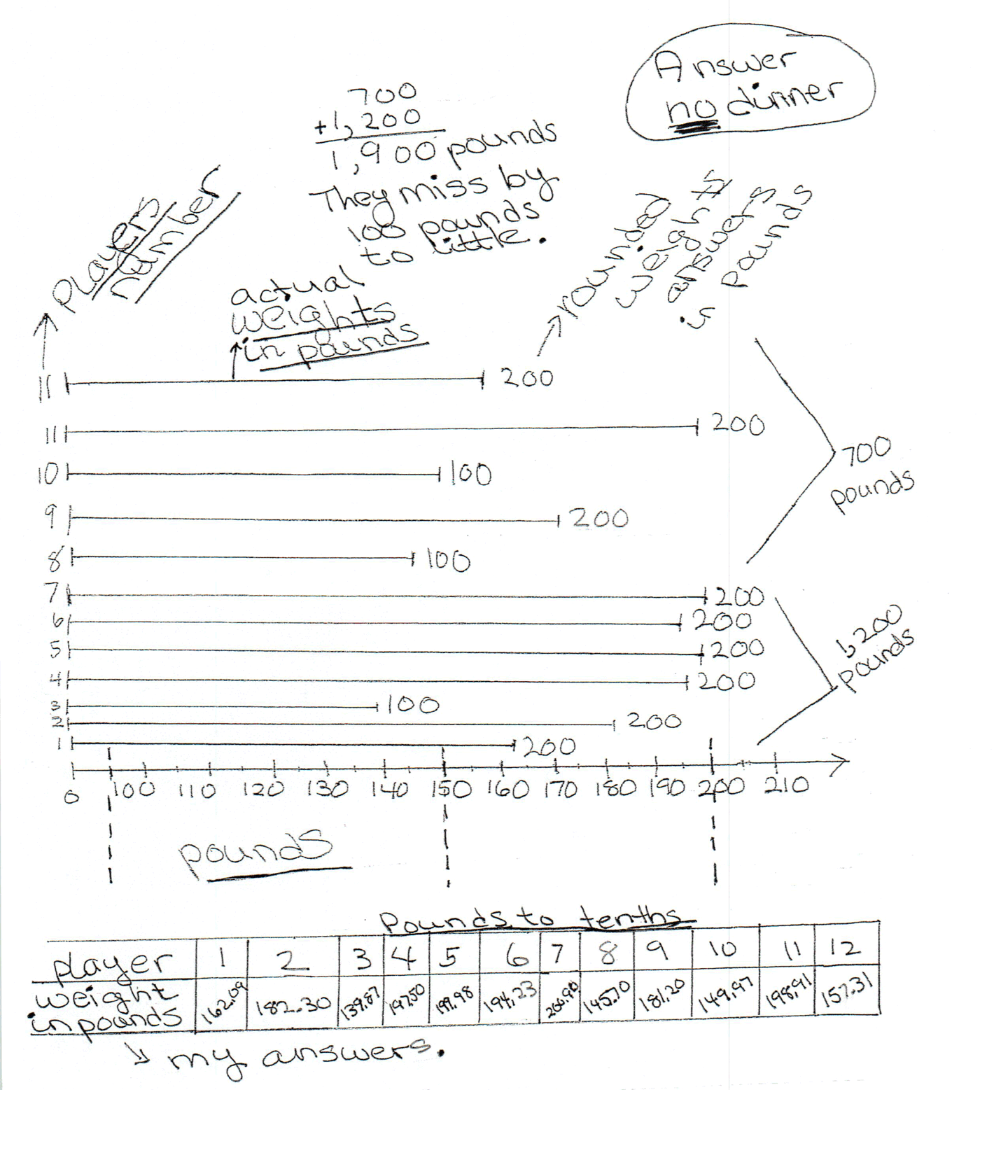This student does not meet the standard.

### Scoring Rationale

#### Apprentice

The student's strategy of using a number line to indicate each football player's weight and that weight rounded to the nearest 100 would work to solve the first part of the task. The student does not correctly notate the ninth player's actual weight. The student omits 100 pounds in the first set of numbers added and 100 pounds in the second set of numbers added. The student's answer, "no dinner," is not correct. The student's strategy of using a table to indicate the weight of each football player rounded to the nearest tenth would work to solve the second part of the task but the student rounds to the nearest hundredth. The student's answers in the weight in pounds row are not correct.

#### Apprentice

The student demonstrates understanding of some of the underlying concepts of the task. The student notes the exact weight of each football player and correctly rounds each weight to the nearest 100. The student shows correct reasoning in adding the rounded weights to compare the sum to 2,000 pounds. It appears that the student rounds each player's weight to the nearest hundredth thinking each weight was rounded to the nearest tenth.

#### Practitioner

The student correctly uses the mathematical terms weights, pounds from the task. The student does not earn credit for the term tenths, as it was not used correctly in the student's rounding/estimations. The student also uses the terms number line, table. The student does not earn credit for the decimal notation in the table as each entry is not correct.

#### Apprentice

The student attempts a connection. The student states, "They miss by 100 pounds to little." This is incorrect as the actual total rounded weight was 2,100 pounds which gives the players an additional 100 pounds of weight.

#### Apprentice

The student attempts a number line that is appropriate for part of the task but is not accurate. The student miscounts the players by using 11 instead of 12 for the twelfth student. The ninth player is not indicated at 181.200 pounds on the number line. The student attempts a table that is appropriate to part of the task but is not accurate. The student rounds each player's weight to the nearest hundredth and not tenth.

#### Apprentice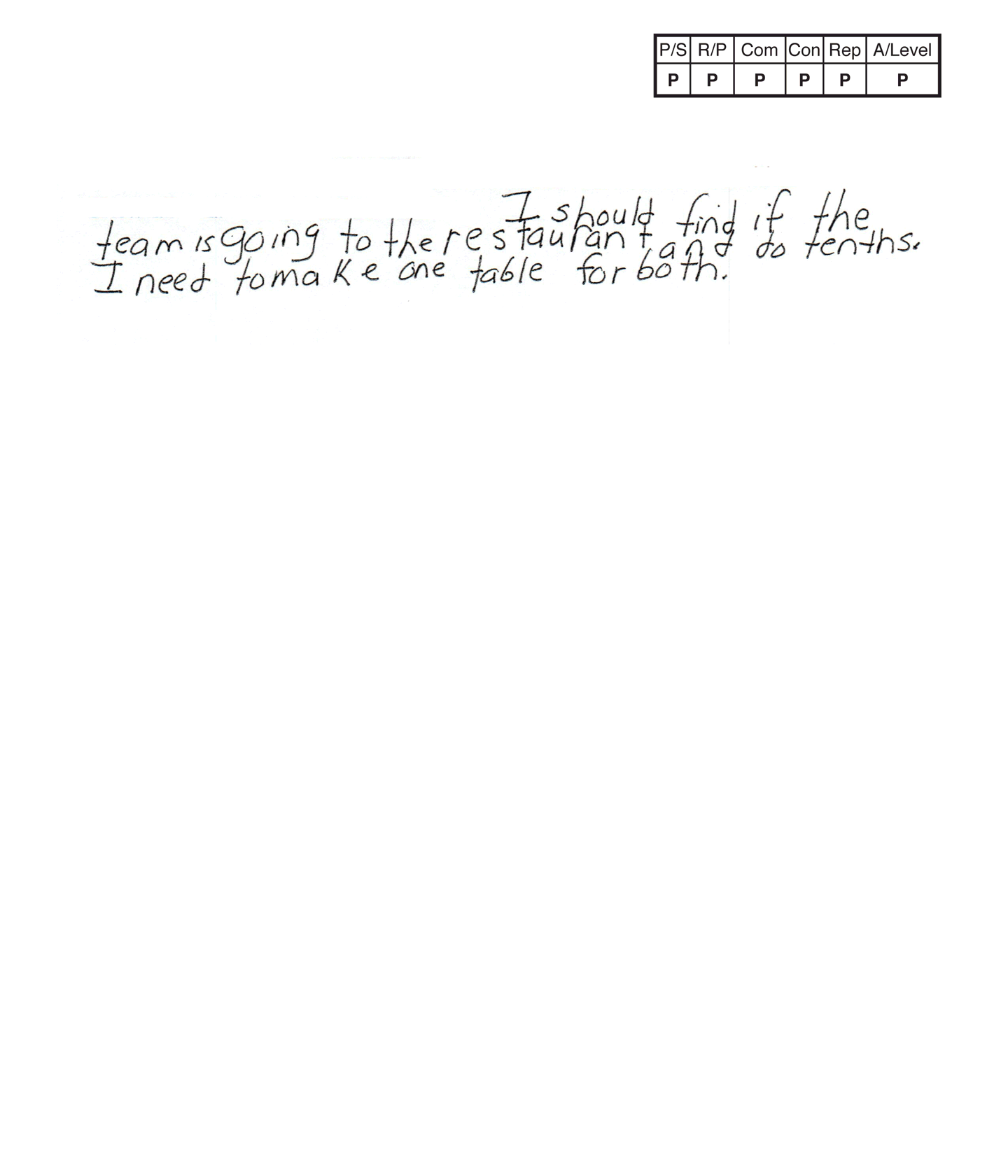,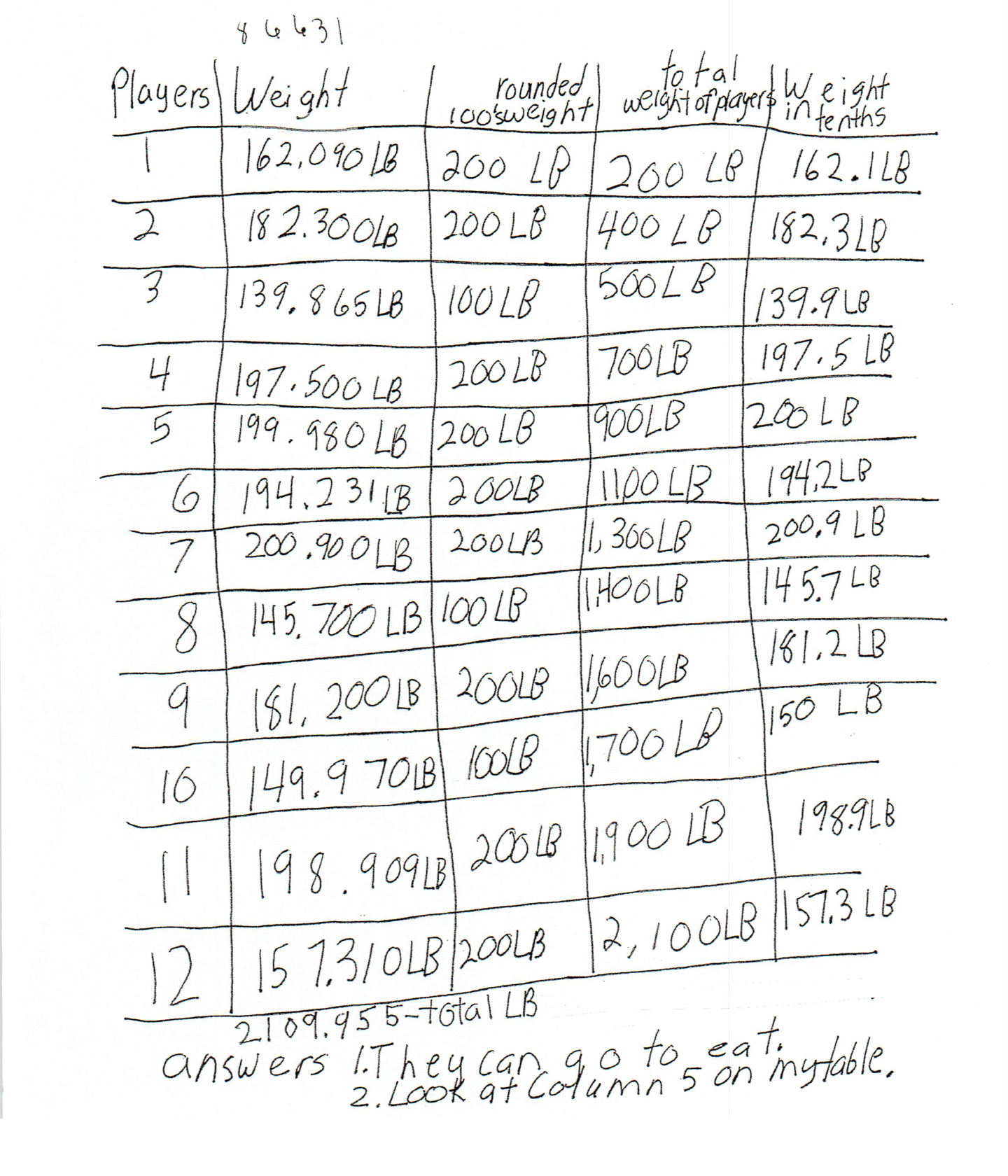,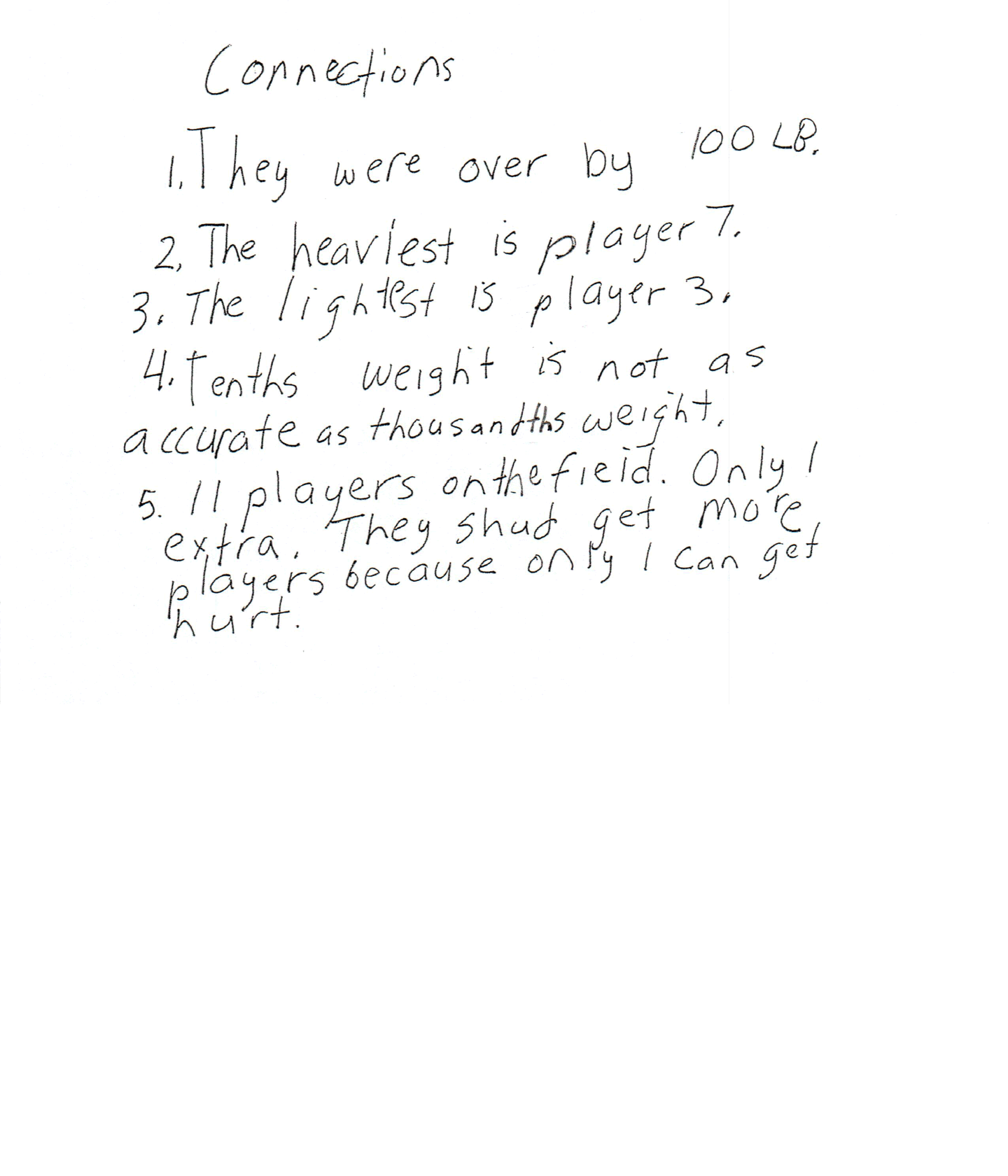This student meets the standard.

### Scoring Rationale

#### Practitioner

The student's strategy of making a table to show the weight of each football player in pounds, the rounded weight to hundreds, the total weight of the players, and weight in tenths, would work to solve the task. The student's answer "1. They can go to eat," and "2. Look at column 5 on my table." are correct.

Note: A student can indicate a column on a table that represents an answer instead of rewriting all the data.

#### Practitioner

The student demonstrates correct reasoning of the underlying concepts of the task. The student correctly rounds each football player's weight, finds the total of the estimated weights and compares that answer to 2,000 pounds. The student finds the actual total pounds of the 12 football players. This might suggest the student is reasoning that this total would confirm that the football players did weigh a total of at least 2,000 pounds. But, the student does not provide enough text to earn the Expert performance level. The student also shows correct reasoning of rounding decimals to the nearest tenth.

#### Practitioner

The student correctly uses the mathematical terms tenths, weight, pounds (LB), total, from the task. The student also uses the terms table, thousandths, more, column. The student correctly uses decimal notation for all football players in the second and fifth columns of their table.

#### Practitioner

The student makes mathematically relevant observations. The student states, "They were over by 100 LB," "The heaviest is player 7," "The lightest is player 3," and, "Tenths weight is not as accurate as thousandths weight."

#### Practitioner

The student's table is appropriate to the task and accurate. All necessary labels are provided and the entered data is correct. The student's "regrouping" numbers for finding the actual sum of the football players' weights are outside the table which is correct.

#### Practitioner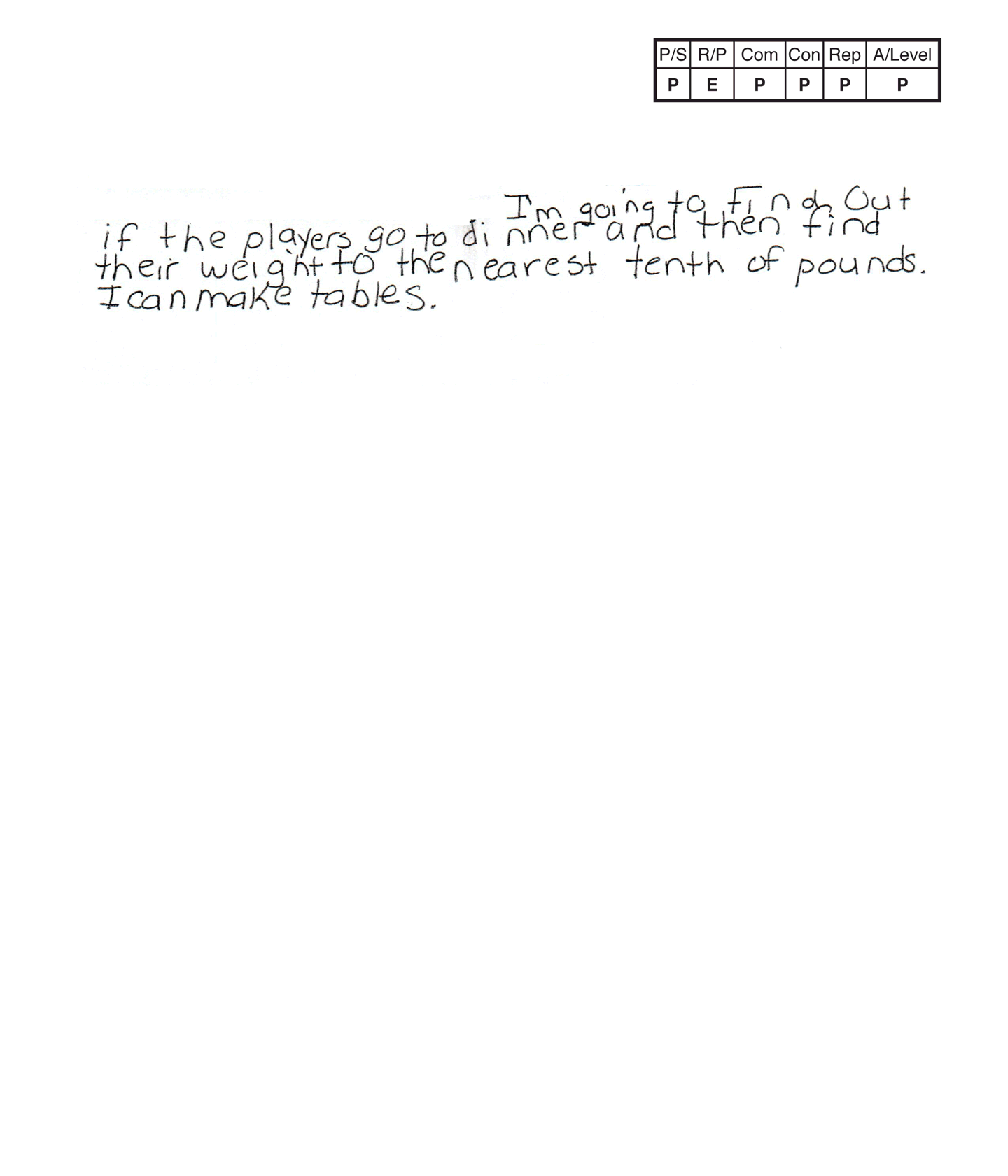,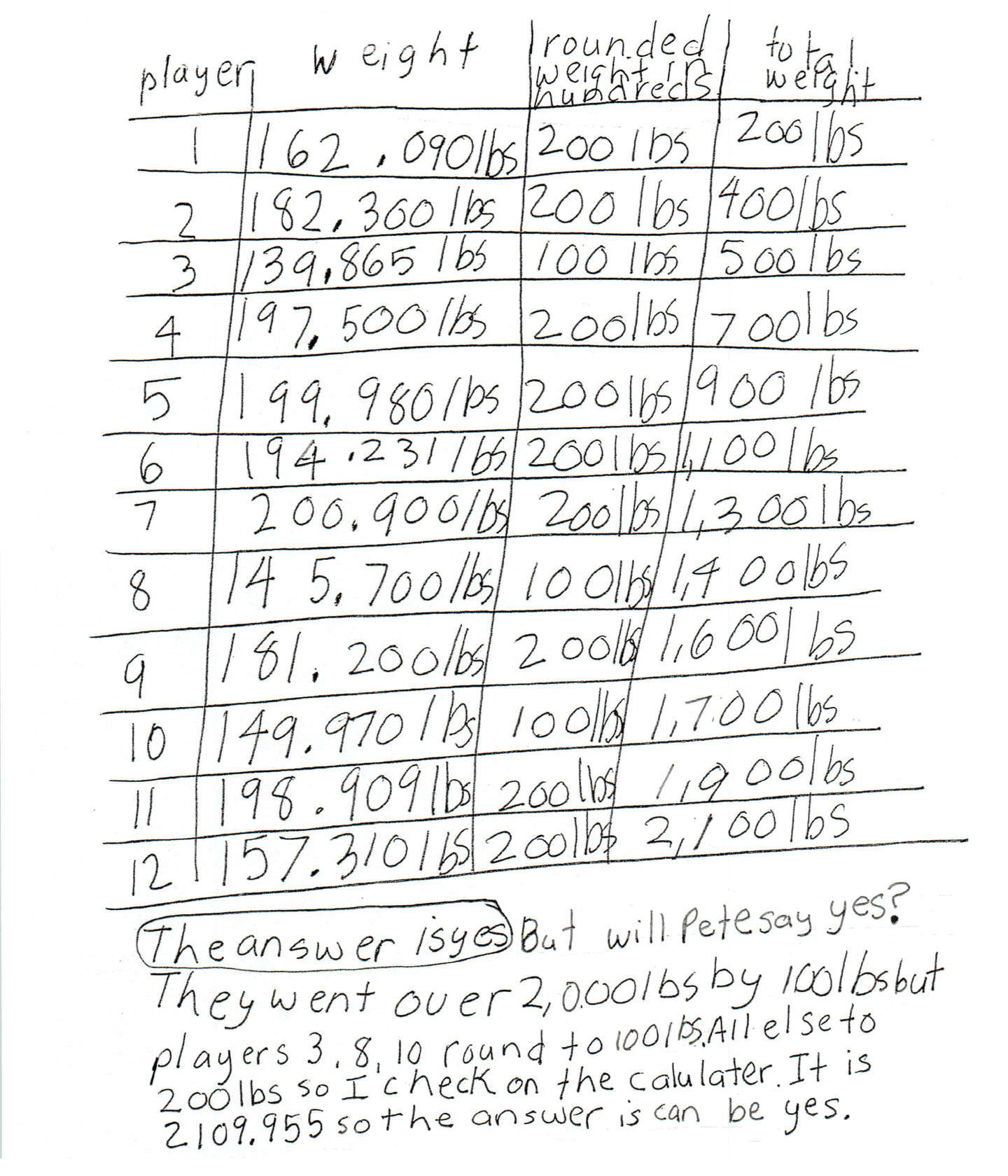,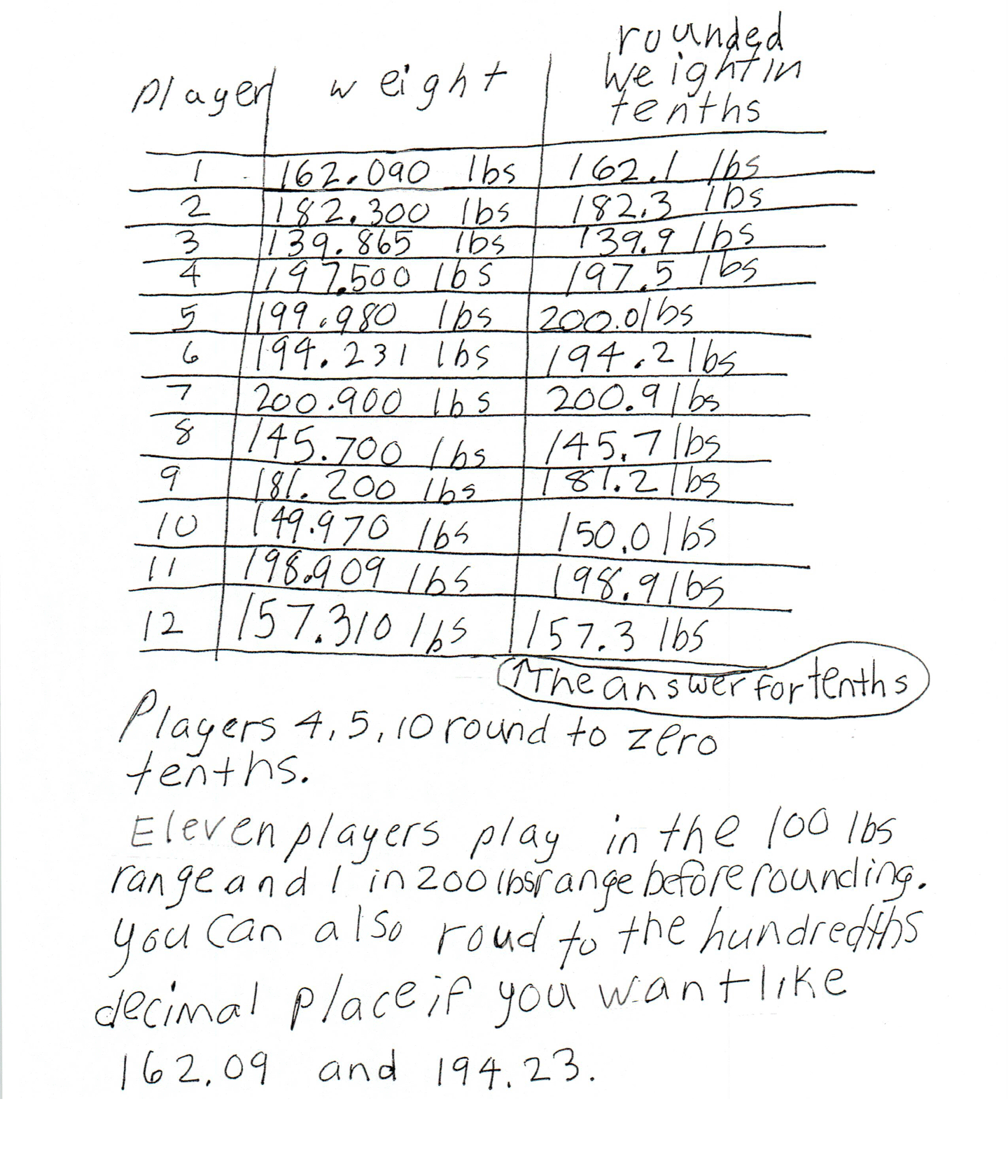This student meets the standard.

### Scoring Rationale

#### Practitioner

The student's strategy of making a table to show the weight of each football player in pounds, the rounded weight to hundreds, the total weight of the players, and comparing that total to 2,000 pounds works to solve the first part of the task. The student's answer, "The answer is yes," is correct. The student's strategy of making a table to show each football player's weight and the rounded weight in tenths, works to solve the second part of the task. The student uses an arrow to indicate that their answers are included in the third column of the table.

#### Expert

The student demonstrates correct reasoning of the underlying concepts of the task. The student correctly rounds the football player weights, finds the total of the estimated weights and compares that answer to 2,000 pounds. The student then considers the phenomenon of rounding/estimation, "... but will Pete say yes?" The student supports their estimation as a correct answer by finding the exact total of the football player weights to make sure the total is at least 2,000 pounds. The student states, "They went over 2,000 lbs by 100 lbs but players 3, 8, 10 round to 100 lbs. All else to 200 lbs so I check on the calulater. It is 2109.955 so the answer is can be yes." The student also shows correct reasoning of rounding decimals to the nearest tenth.

#### Practitioner

The student correctly uses the mathematical terms weight, tenth, pounds (lbs), hundreds from the task. The student also uses the terms tables, range, hundredths, decimal place. The student correctly uses decimal notation for all football player weights in the two tables.

#### Practitioner

The student makes mathematically relevant observations. The student states, "Eleven players play in the 100 lbs range and 1 in 200 lbs range before rounding," and "You can also roud to the hundredths decimal place if you want like 162.09 and 194.23."

#### Practitioner

The student's two tables are appropriate to the task and accurate. All necessary labels are provided and the entered data is correct.

#### Practitioner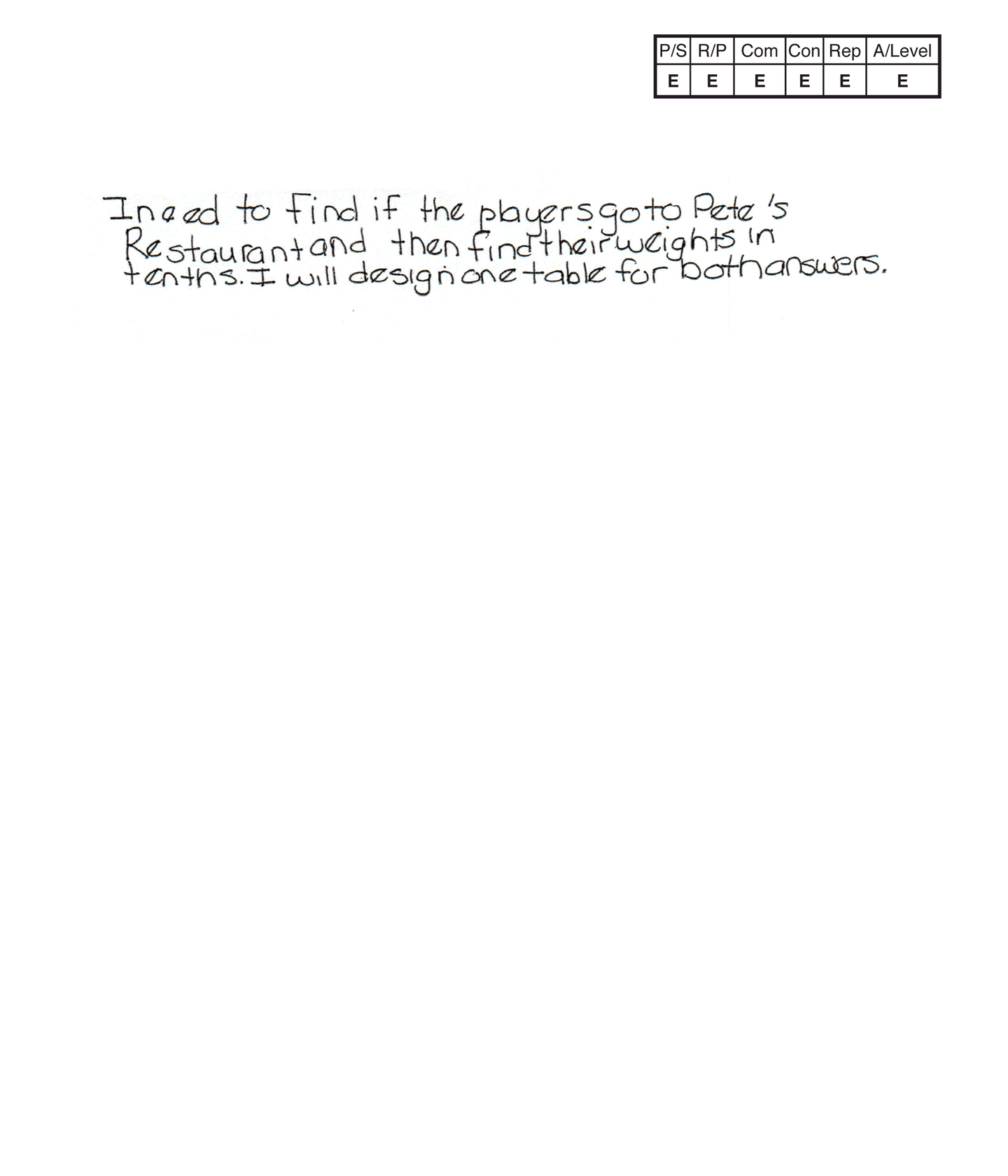,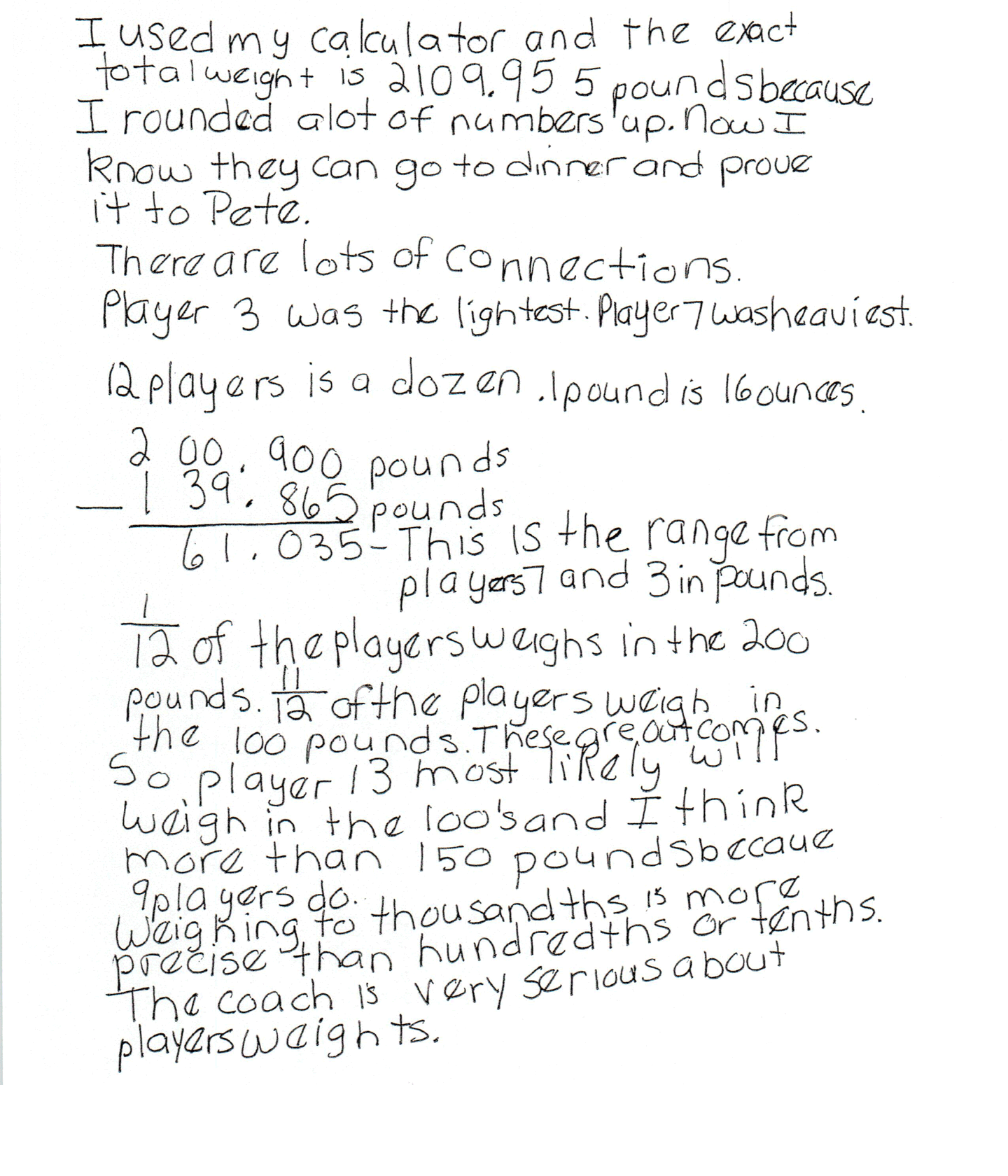,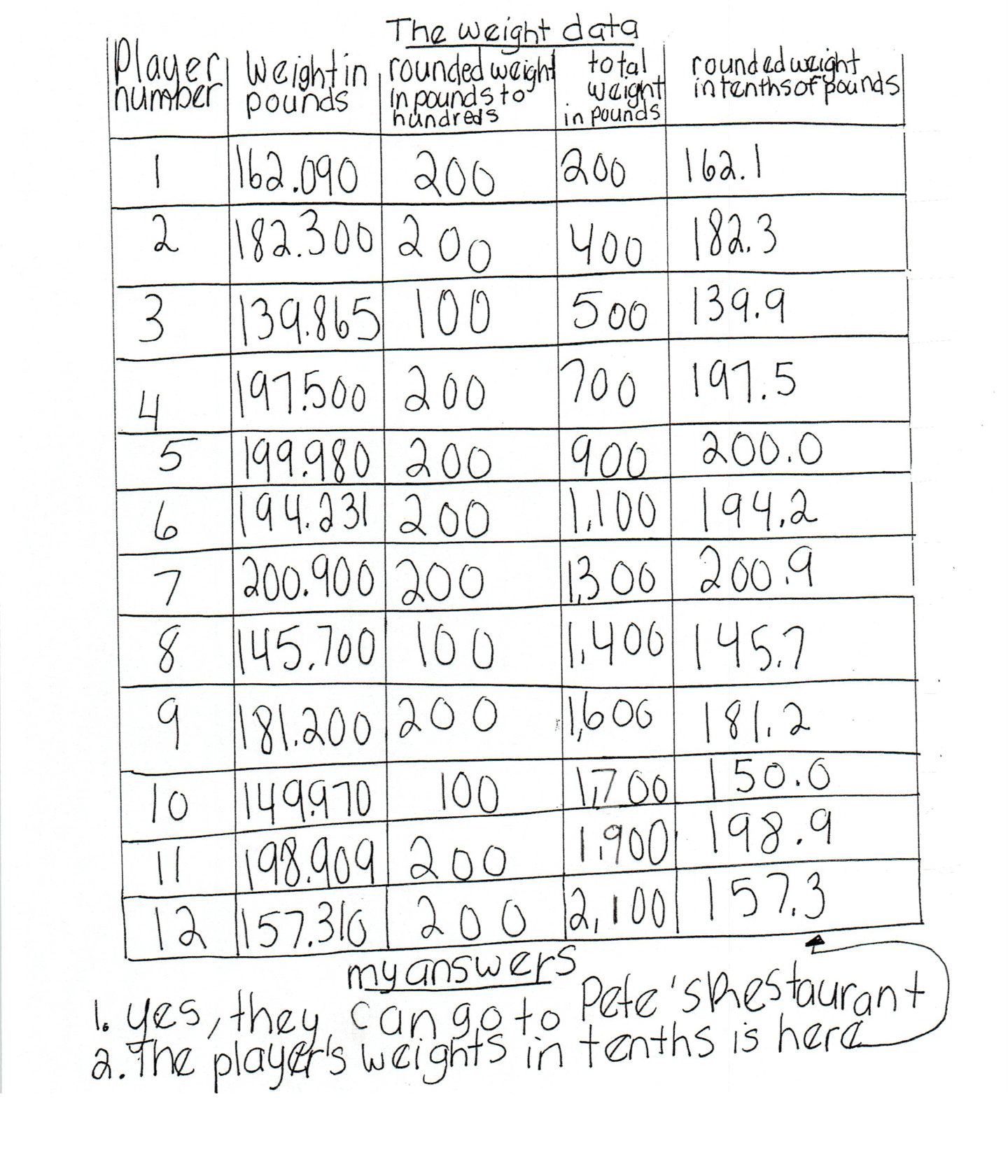,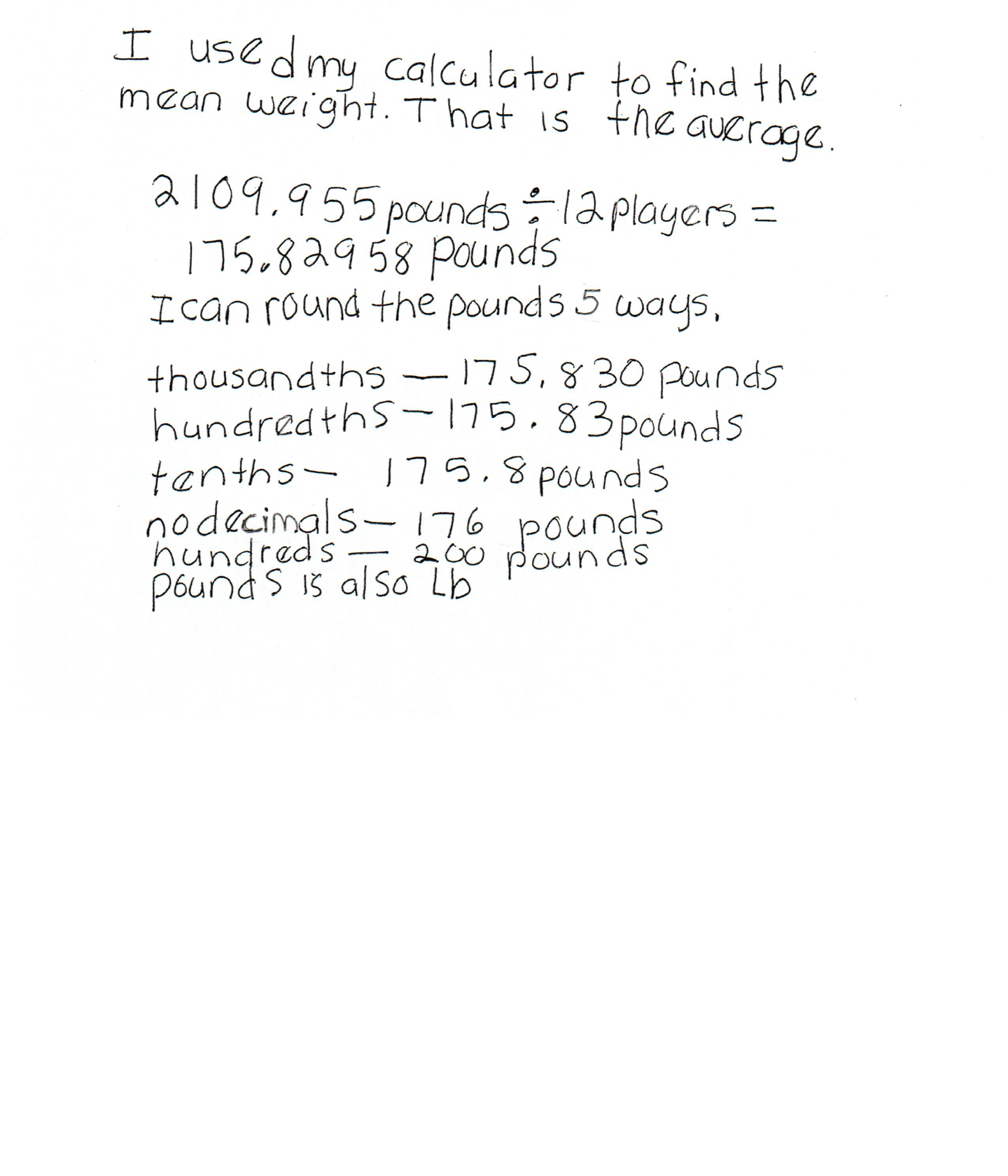This student exceeds the standard.

### Scoring Rationale

#### Expert

The student's strategy of making a table to show the weight of each football player in pounds, the rounded weight to hundreds, the total weight of the players, and comparing that total to 2,000 pounds works to solve the first part of the task. The student's fifth column on their table includes the rounded weight in tenths of pounds data to answer the second part of the task. The student's answers "1. Yes, they can go to Pete's Restaurant," and, "2. The player's weights in tenths is here" (with an arrow pointing to the fifth column), are correct. The student brings prior knowledge of statistics to their solution.

#### Expert

The student demonstrates correct reasoning of the underlying concepts of the task. The student correctly rounds the football player weights, finds the total of the estimated weights and compares that answer to 2,000 pounds. The student then considers the phenomenon of rounding/estimation The student supports their estimation as a correct answer by finding the exact total of the football player weights to make sure the total is at least 2,000 pounds. The student states, "I used my calculator and the exact total weight is 2109.955 pounds because I rounded a lot of numbers up. Now I know they can go to dinner and prove it to Pete." The student also shows correct reasoning of rounding decimals to the nearest tenth as well as thousandths and hundreds. The student applies the concepts of statistics, including ratio, to the task.

#### Expert

The student correctly uses the mathematical terms weights, tenths, pounds, total, hundreds from the task. The student also uses the terms table, data, dozen, ounces, range, outcomes, most likely, more than, thousandths, mean, average, hundredths, decimal, number. The student correctly uses the mathematical notation 1/12, 11/12. The student correctly uses decimal notation in both their table and text.

#### Expert

The student makes mathematically relevant Practitioner connections. The student states, "Player 3 was the lightest. Player 7 was heaviest," "12 players is a dozen," and "1 pound is 16 ounces." The student makes the Expert connections, "61.035 - This is the range from players 7 and 3 in pounds," "1/12 of the players weighs in the 200 pounds," "11/12 of the players weigh in the 100 pounds, These are outcomes," "So player 13 most likely will weigh in the 100's and I think more than 150 pounds because 9 players do." The student states, "Weighing to thousandths is more precise than hundredths or tenths. The coach is very serious about players weights." The student also states, "I used my calculator to find the mean weight. That is the average." The student accurately divides to find 175.82958 as the average and then rounds this answer five ways. The student states, "thousandths - 175.830 pounds, hundredths - 175.83 pounds, tenths - 175.8 pounds, no decimals - 176 pounds, hundreds - 200 pounds."

#### Expert

The student's table is both appropriate to the task and accurate. All necessary labels are provided and the entered data is correct. The student uses the data on their table to link to statistics by finding the range, outcomes, what is most likely, and average.